International
Tables for
Crystallography
Volume B
Reciprocal space
Edited by U. Shmueli

International Tables for Crystallography (2006). Vol. B, ch. 1.1, pp. 5-7   | 1 | 2 |

## Section 1.1.4. Tensor-algebraic formulation

U. Shmuelia*

aSchool of Chemistry, Tel Aviv University, 69 978 Tel Aviv, Israel
Correspondence e-mail: ushmueli@post.tau.ac.il

### 1.1.4. Tensor-algebraic formulation

| top | pdf |

The present section summarizes the tensor-algebraic properties of mutually reciprocal sets of basis vectors, which are of importance in the various aspects of crystallography. This is not intended to be a systematic treatment of tensor algebra; for more thorough expositions of the subject the reader is referred to relevant crystallographic texts (e.g. Patterson, 1967; Sands, 1982), and other texts in the physical and mathematical literature that deal with tensor algebra and analysis.

Let us first recall that symbolic vector and matrix notations, in which basis vectors and coordinates do not appear explicitly, are often helpful in qualitative considerations. If, however, an expression has to be evaluated, the various quantities appearing in it must be presented in component form. One of the best ways to achieve a concise presentation of geometrical expressions in component form, while retaining much of their `transparent' symbolic character, is their tensor-algebraic formulation.

#### 1.1.4.1. Conventions

| top | pdf |

We shall adhere to the following conventions:

 (i) Notation for direct and reciprocal basis vectors: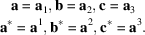Subscripted quantities are associated in tensor algebra with covariant, and superscripted with contravariant transformation properties. Thus the basis vectors of the direct lattice are represented as covariant quantities and those of the reciprocal lattice as contravariant ones. (ii) Summation convention: if an index appears twice in an expression, once as subscript and once as superscript, a summation over this index is thereby implied and the summation sign is omitted. For example,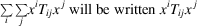since both i and j conform to the convention. Such repeating indices are often called dummy indices. The implied summation over repeating indices is also often used even when the indices are at the same level and the coordinate system is Cartesian; there is no distinction between contravariant and covariant quantities in Cartesian frames of reference (see Chapter 3.3). (iii) Components (coordinates) of vectors referred to the covariant basis are written as contravariant quantities, and vice versa. For example,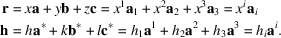#### 1.1.4.2. Transformations

| top | pdf |

A familiar concept but a fundamental one in tensor algebra is the transformation of coordinates. For example, suppose that an atomic position vector is referred to two unit-cell settings as follows: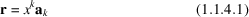and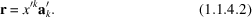Let us multiply both sides of (1.1.4.1)and (1.1.4.2), on the right, by the vectors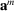, m = 1, 2, or 3, i.e. by the reciprocal vectors to the basis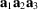. We obtain from (1.1.4.1)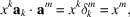where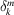is the Kronecker symbol which equals 1 when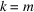and equals zero if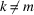, and by comparison with (1.1.4.2)we have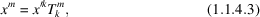where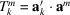is an element of the required transformation matrix. Of course, the same transformation could have been written as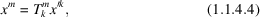where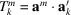.

A tensor is a quantity that transforms as the product of coordinates, and the rank of a tensor is the number of transformations involved (Patterson, 1967; Sands, 1982). E.g. the product of two coordinates, as in the above example, transforms from the a′ basis to the a basis as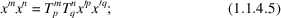the same transformation law applies to the components of a contravariant tensor of rank two, the components of which are referred to the primed basis and are to be transformed to the unprimed one: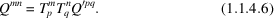#### 1.1.4.3. Scalar products

| top | pdf |

The expression for the scalar product of two vectors, say u and v, depends on the bases to which the vectors are referred. If we admit only the covariant and contravariant bases defined above, we have four possible types of expression: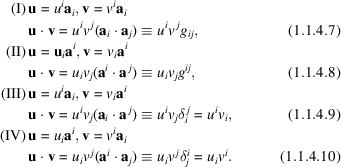(i) The sets of scalar products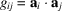(1.1.4.7)and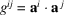(1.1.4.8)are known as the metric tensors of the covariant (direct) and contravariant (reciprocal) bases, respectively; the corresponding matrices are presented in conventional notation in equations (1.1.3.11)and (1.1.3.13). Numerous applications of these tensors to the computation of distances and angles in crystals are given in Chapter 3.1. (ii) Equations (1.1.4.7)to (1.1.4.10)furnish the relationships between the covariant and contravariant components of the same vector. Thus, comparing (1.1.4.7)and (1.1.4.9), we have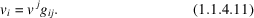Similarly, using (1.1.4.8)and (1.1.4.10)we obtain the inverse relationship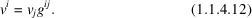The corresponding relationships between covariant and contravariant bases can now be obtained if we refer a vector, say v, to each of the bases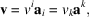and make use of (1.1.4.11)and (1.1.4.12). Thus, e.g.,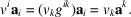Hence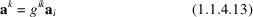and, similarly,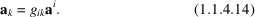(iii) The tensorsand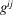are symmetric, by definition. (iv) It follows from (1.1.4.11)and (1.1.4.12)or (1.1.4.13)and (1.1.4.14)that the matrices of the direct and reciprocal metric tensors are mutually inverse, i.e.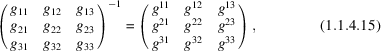and their determinants are mutually reciprocal.

#### 1.1.4.4. Examples

| top | pdf |

There are numerous applications of tensor notation in crystallographic calculations, and many of them appear in the various chapters of this volume. We shall therefore present only a few examples.

### References

International Tables for Crystallography (2004). Vol. C. Mathematical, physical and chemical tables, edited by E. Prince. Dordrecht: Kluwer Academic Publishers.
Patterson, A. L. (1967). In International tables for X-ray crystallography, Vol. II. Mathematical tables, edited by J. S. Kasper & K. Lonsdale, pp. 5–83. Birmingham: Kynoch Press.
Sands, D. E. (1982). Vectors and tensors in crystallography. New York: Addison-Wesley.
Schomaker, V. & Trueblood, K. N. (1968). On the rigid-body motion of molecules in crystals. Acta Cryst. B24, 63–76.